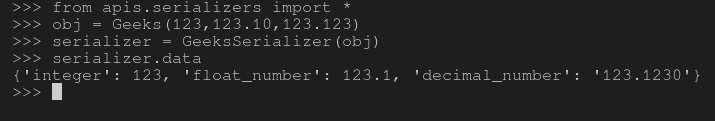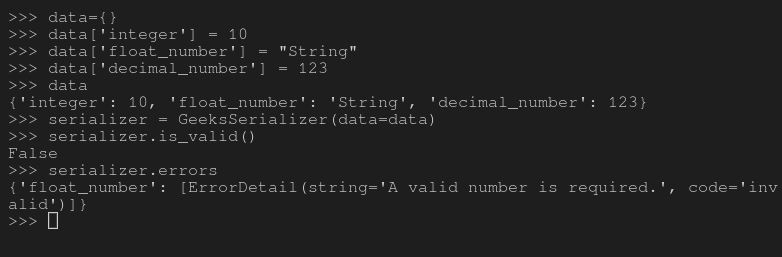# Numeric fields in serializers – Django REST Framework

In Django REST Framework the very concept of Serializing is to convert DB data to a datatype that can be used by javascript. Every serializer comes with some fields (entries) which are going to be processed. For example if you have a class with name Employee and its fields as Employee_id, Employee_name, is_admin, etc. Then, you would need AutoField, CharField and BooleanField for storing and manipulating data through Django. Similarly, serializer also works with same principle and has fields that are used to create a serializer.
This article revolves around Numeric Fields in Serializers in Django REST Framework. There are three major fields – IntegerField, FloatField and DecimalField.

#### IntegerField

IntegerField is basically a integer field that validates the input against Python’s int instance.It is same as IntegerField – Django Models
It has the following arguments –

• max_value Validate that the number provided is no greater than this value.
• min_value Validate that the number provided is no less than this value.

Syntax –

`field_name = serializers.IntegerField(*args, **kwargs)`

#### FloatField

FloatField is basically a float field that validates the input against Python’s float instance.It is same as FloatField – Django Models
It has the following arguments –

• max_value Validate that the number provided is no greater than this value.
• min_value Validate that the number provided is no less than this value.

Syntax –

`field_name = serializers.FloatField(*args, **kwargs)`

#### DecimalField

DecimalField is basically a decimal field that validates the input against Python’s decimal instance.It is same as DecimalField – Django Models
It has the following arguments –

• max_digits The maximum number of digits allowed in the number. It must be either None or an integer greater than or equal to decimal_places.
• decimal_places The number of decimal places to store with the number.
• max_value Validate that the number provided is no greater than this value.
• min_value Validate that the number provided is no less than this value.
• localize Set to True to enable localization of input and output based on the current locale.

Syntax –

`field_name = serializers.DecimalField(*args, **kwargs)`

#### How to use Numeric Fields in Serializers ?

To explain the usage of Numeric Fields, let’s use the same project setup from – How to Create a basic API using Django Rest Framework ?
Now that you have a file called serializers in your project, let’s create a serializer with IntegerField, FloatField and DecimalField as the fields.

## Python3

 `# import serializer from rest_framework` `from` `rest_framework ``import` `serializers`   `class` `Geeks(``object``):` `    ``def` `__init__(``self``, integer, float_number, decimal_number):` `        ``self``.integer ``=` `integer` `        ``self``.float_number ``=` `float_number` `        ``self``.decimal_number ``=` `decimal_number`   `# create a serializer` `class` `GeeksSerializer(serializers.Serializer):` `    ``# initialize fields` `    ``integer ``=` `serializers.IntegerField()` `    ``float_number ``=` `serializers.FloatField()` `    ``decimal_number ``=` `serializers.DecimalField()`

Now let us create some objects and try serializing them and check if they are actually working, Run, –

`Python manage.py shell`

Now, run following python commands in the shell

```# import everything from serializers
>>> from apis.serializers import *

# create a object of type Geeks
>>> obj = Geeks(123, 123.10, 123.123)

# serialize the object
>>> serializer = GeeksSerializer(obj)

# print serialized data
>>> serializer.data
{'integer': 123, 'float_number': 123.1, 'decimal_number': '123.1230'}```

Here is the output of all these operations on terminal –#### Validation on Numeric Fields

Note that prime motto of these fields is to impart validations, such as IntegerField validates the data to Python’s int only. Let’s check if these validations are working or not –

```# Create a dictionary and add invalid values
>>> data={}
>>> data['integer'] = 10
>>> data['float_number'] = "String"
>>> data['decimal_number'] = 123

# dictionary created
>>> data
{'integer': 10, 'float_number': 'String', 'decimal_number': 123}

# deserialize the data
>>> serializer = GeeksSerializer(data=data)

# check if data is valid
>>> serializer.is_valid()
False

# check the errors
>>> serializer.errors
{'float_number': [ErrorDetail(string='A valid number is required.',
code='invalid')]}
}```

Here is the output of these commands which clearly shows email and phone_number as invalid –Validations are part of Deserialization and not serialization. As explained earlier, serializing is process of converting already made data into another data type, so there is no requirement of these default validations out there. Deserialization requires validations as data needs to be saved to database or any more operation as specified. So if you serialize data using these fields that would work.

## Core arguments in serializer fields

.math-table { border-collapse: collapse; width: 100%; } .math-table td { border: 1px solid #5fb962; text-align: left !important; padding: 8px; } .math-table th { border: 1px solid #5fb962; padding: 8px; } .math-table tr>th{ background-color: #c6ebd9; vertical-align: middle; } .math-table tr:nth-child(odd) { background-color: #ffffff; }

Whether you're preparing for your first job interview or aiming to upskill in this ever-evolving tech landscape, GeeksforGeeks Courses are your key to success. We provide top-quality content at affordable prices, all geared towards accelerating your growth in a time-bound manner. Join the millions we've already empowered, and we're here to do the same for you. Don't miss out - check it out now!